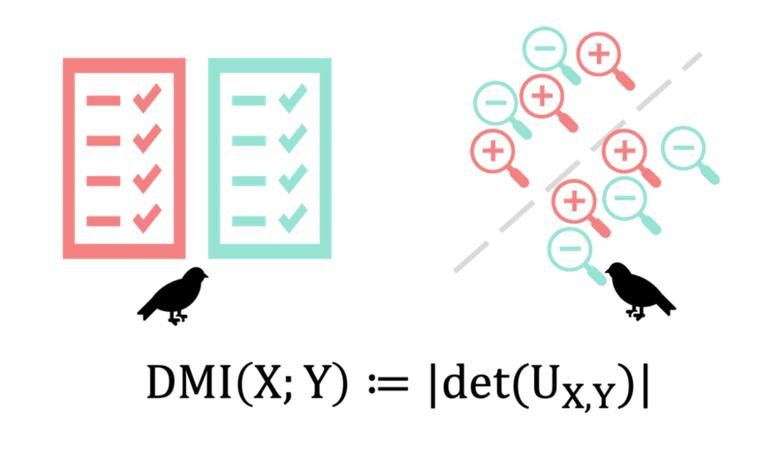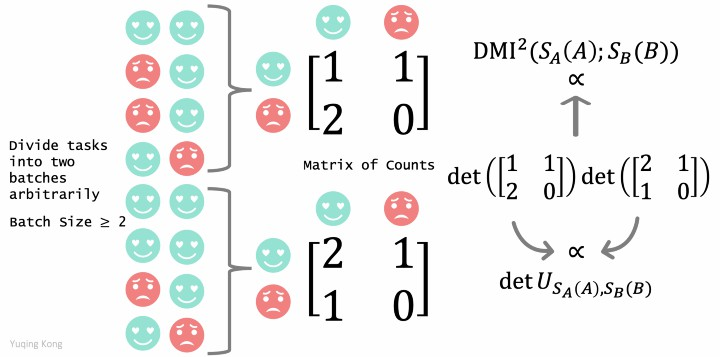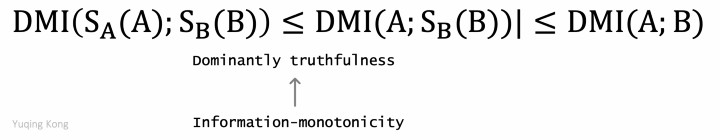News
News

## Determinant based Mutual Information

kill two birds with one stoneTwo birds:

1. Alice and Bob are rating 10 online products, how to reward them such that they are encouraged to tell the truth?
2. We want to train a medical-image-classifier. However, the given labels are noisy. How to design the loss function such that the training process is robust to the noise?

For the first question, the challenge is that Alice and Bob' ratings are subjective thus we cannot verify it. One way to pay Alice is to use Bob' ratings as a reference. For example, a natural attempt is to pay Alice and Bob by the number of agreements they have. However, honest Alice and honest Bob may have very different opinions. Moreover, they can collude to give all products the highest ratings to obtain the highest reward.

This question is called multi-task peer prediction. Peer prediction is initially proposed by Miller, Resnick, Zeckhauser 2004. Miller, Resnick, Zeckhauser 2004 consider a single-task setting where the participants are assigned a single task (e.g. Do you like Panda express?). The multi-task setting (e.g. the multiple products rating setting) is proposed by Dasgupta and Gosh 2013. In the multi-task setting, a series of works (e.g. Shnayder, Agarwal, Frongillo, Parkes 2016, Kong and Schoenebeck 2019, Liu, Wang, Chen 2020) successfully develop reward schemes where truth-telling is the best for each participant, regardless of other participants' strategy (dominantly truthful). However, they require the participants to perform an infinite number of tasks (e.g. rating an infinite number of tasks).

For the second question, the challenge is very similar to the first one when we use noisy labels as a reference to evaluate the classifier. Cross-entropy loss does not work here since if we use cross-entropy, we are training the classifier to predict the noisy labels rather than the true labels. This question is called noisy learning problem and studied by many works (e.g. Natarajan et al. 2013).

One stone:

The stone is a new mutual information measure, Determinant based Mutual Information (DMI), proposed by Kong 2020 (Talk). The high-level idea is:

1. reward Alice and Bob the Determinant based Mutual Information between their ratings.
1. evaluate the classifier by the Determinant based Mutual Information between the classifier's outputs and the noisy labels. The training process returns the classifier with the highest evaluation.

Mutual Information

Intuitively, mutual information measures the amount of information shared by two random variables X, Y. For example, two independent random variables have zero mutual information. Two highly correlated random variables have high mutual information. The first definition of mutual information is proposed by Shannon. Shannon Mutual Information is defined as the KL divergence between the joint distribution over X, Y and the product of marginal distributions. One important property Shannon Mutual Information has is information-monotonicity:

When X and M(Y) are independent conditioning on Y, the mutual information between X and M(Y) is less than the mutual information between X and Y.

This property means that any data processing method operated on one side will decrease the mutual information.

Determinant based Mutual Information (DMI)

DMI is defined as the absolute value of the determinant of the joint distribution matrix.Determinant based Mutual Information

Like Shannon mutual information, Determinant based Mutual Information is symmetric, non-negative and satisfies information-monotonicityDMI is information-monotone.

Two additional special properties of DMI are

1. The square of DMI is a polynomial thus can be estimated unbiasedly by a finite number of samples.
2. Relative invariance: DMI(X;M(Y))=DMI(X;Y)|det(M)|Two special properties of DMI

These two special properties allow DMI has applications in both peer prediction and noisy learning setting.

In the multi-task peer prediction setting, unlike previous mechanisms which require an infinite number of tasks to be dominantly truthful, DMI induces a simple dominantly truthful mechanism, DMI-Mechanism, which only requires ≥2C number of tasks, where C is the number of rating options.

DMI-Mechanism:

We divide the products into two batches arbitrarily to guarantee that each batch's size is greater than the number of rating options. For example, if it is a binary choice rating, like/dislike, each batch's size should be greater than 2. For each batch, compute the empirical counts between Alice and Bob's ratings within the batch. Finally, return the product of the determinant of the batches' empirical counts matrices.DMI-Mechanism

Analysis of DMI-Mechanism:

We assume the rating tasks are similar and independent, then Alice and Bob's honest ratings can be seen as i.i.d. samples from joint random variables (A,B).

We assume that Alice (Bob) performs the same (possibly random) strategy for all tasks. Then Alice's strategy S_A can be seen as an operation on A and Bob's strategy S_B can be seen as an operation on B. Then Alice and Bob's ratings can be seen as i.i.d. samples from joint random variables (S_A(A),S_B(B)).

We also show that In expectation, each counts matrix's determinant is proportional to the determinant of the joint distribution over (S_A(A),S_B(B)).

Thus, we reward Alice and Bob the square of DMI between their ratings.Due to information-monotonicity of DMI, truth-telling is the best for each participant, regardless of other participants' strategy (dominantly truthful).The implementation of DMI-Mechanism only requires each batch size ≥ C to guarantee that the expectation of the reward is not always zero. Previous work essentially designs the reward by Shannon mutual information or other similar mutual information family designed by convex functions. Therefore, in those mechanisms, the dominantly truthful property requires an infinite number of tasks.

DMI-Scorer:

In the noisy learning setting, for each classifier h, we compute the empirical joint frequency matrix between h's output and labels; return the absolute value of the matrix's determinant as evaluation for h. The training process returns the h with the highest evaluation.DMI-Scorer

Analysis of DMI-Scorer:

In the noisy learning setting, if the empirical frequency estimation is sufficiently good, the classifier h is evaluated via DMI between h's outputs and the noisy labels, i.e., DMI(Classifier h; Noise(Ground truth Labels)) where Noise(Ground-truth labels) is the noisy labels which are obtained by performing a noise-operation on the ground truth labels.

When we assume that the noise is independent of the classifier, the relative invariance property of DMI implies that the evaluation is robust to noise, that is, If h has a higher DMI with ground truth labels than h', it will also have a higher DMI with noisy labels than h'.To apply DMI-Scorer in noisy learning in practice, Xu*, Cao*, Kong, Wang 2019 propose a loss function which is the negative logarithm of DMI-Scorer.

Limitation:

The biggest limitation of DMI is that it vanishes not only when X and Y are independent, but also when the joint distribution matrix is degenerate. In the asymmetric setting or high dimensional setting when the number of rating options/label types is high, the direct DMI may not be applicable. One possible solution is to compress both sides into a small discrete set and compute DMI of the compressed set. But this will not preserve the properties of DMI.

Summary:

Determinant based Mutual Information is a new definition of mutual information, which not only shares some important properties with Shannon Mutual Information, but also has two additional special properties. Interestingly, these two properties make DMI 1) significantly reduce the task complexity in the multi-task peer prediction setting; 2) induce a fully noise-robust loss function. Later we will talk about geometric interpretation of DMI and generalization of DMI.

Thanks to Paul Resnick